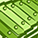# QlikView App Dev

Discussion Board for collaboration related to QlikView App Development.

Announcements
Our next Qlik Insider session will cover new key capabilities. Join us August 11th REGISTER TODAY
cancel
Showing results for
Did you mean:Not applicable

## Variables

Hi there, this is the part in my script that creates the set analysis part:

Let vCurrentCycle = Peek('Cycle Calendar Period',0,'Cycles');

Let vPrevMonthCycle = vCurrentCycle-1;

let vPrevYearCycle = vCurrentCycle-100;

Let vEFT_VOL_CP = '{<[Cycle Calendar Period]={\$(vCurrentCycle)}>}';

Let vEFT_VOL_PP = '{<[Cycle Calendar Period]={\$(vPrevYearCycle)}>}';

Let vEFT_VOL_CP = '{<[Cycle Calendar Period]={\$(vPrevMonthCycle)}>}';

in my equation i'm trying this:

=sum( {\$<\$(vEFT_VOL_CP)>}  [EFT Volume]), i have tried the following variations as well.

=sum( {\$<'\$(vEFT_VOL_CP)'>}  [EFT Volume])

=sum( {\$<{'\$(vEFT_VOL_CP)'}>}  [EFT Volume])

what is it i'm doing wrong.

1 Solution

Accepted SolutionsNot applicable
Author

i figured out my error, i created 2 variables with the exact same name

5 RepliesSpecialist II

maybe this

=sum('\$(vEFT_VOL_CP)'  [EFT Volume] )Not applicable
Author

Error: Error in expression:

')' expectedMVP

Hi Stephan,

Try this,

Let vEFT_VOL_CP = '{\$<[Cycle Calendar Period]={\$(vCurrentCycle)}>}';

Let vEFT_VOL_PP = '{\$<[Cycle Calendar Period]={\$(vPrevYearCycle)}>}';

Let vEFT_VOL_CP = '{\$<[Cycle Calendar Period]={\$(vPrevMonthCycle)}>}';

=Sum(\$(vEFT_VOL_CP) [EFT Volume])Not applicable
Author

i figured out my error, i created 2 variables with the exact same nameMVP

Glad you found the mistake.I thought it was a typo. Have a nice day!!Community Browser